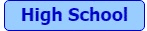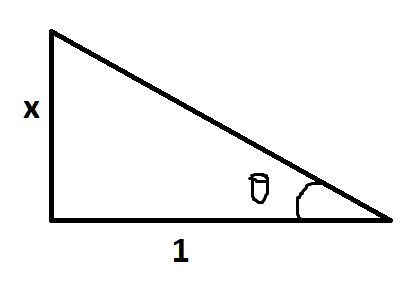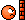# Math Challenge - August 2019

• Challenge
• fresh_42
• Featured
In summary, the maximum value of ff with f(x)=xae2a−xf(x)=xae2a−x is minimal for positive numbers a≥1a≥1. The equation of the curve is y=5x−4y=5x−4 and the flight paths will be three straight lines intersecting at (−2,1)(−2,1). The smallest field extension of FqFq containing an nn-th root of unity is FqnFqn. The triple (a,b,c)(a,b,c) cannot exist in Z3Z3. The function f(x)f(x) is not differentiable at x=1/2x=1/2. This function is strictly increasing or decreasing

#### fresh_42

Mentor
2022 Award
Questions

1.
(solved by @Pi-is-3 )The maximum value of ##f## with ##f(x) = x^a e^{2a - x}## is minimal for which values of positive numbers ##a## ?

2. (solved by @KnotTheorist ) Find the equation of a curve such that ##y''## is always ##2## and the slope of the tangent line is ##10## at the point ##(2,6)##.

3. (solved by @nuuskur ) Let ##F_q## be the finite field with ##q## elements and ##n \geq 1## with ##(n,q)=1##. What is the smallest field extension of ##F_q## containing an ##n##-th root of unity?

4. (solved by @nuuskur ) Show that there is no infinite countable ##\sigma##-algebra.

5. (solved by @nuuskur ) Classify all groups of order ##6,8,## and ##12##.

6. (solved by @nuuskur ) Let ##G## be a group of order ##2m## with ##m>1## an odd number. Show that ##G## is not simple, i.e. has at least one non trivial normal subgroup.

7. (a) (solved by @nuuskur ) Show that a group ##G## is abelian if and only the map ##g \mapsto g^{-1}## is a group homomorphism.
7. (b) (solved by @nuuskur ) Suppose that ##G## is a finite group and that we have ##\sigma \in \operatorname{Aut}(G)## with the neutral element ##e## as unique fixed point. If ##\sigma^2=\operatorname{id}_G##, prove that ##G## is abelian.
Hint: Prove that every element of ##G## can be written in the form ##x^{-1} \sigma(x)## and apply ##\sigma## to such an expression.
(Credits will only be given for solving both subquestions.)

8. (solved by @Pi-is-3 ) Show that there is no triple ##(a,b,c) \in \mathbb{Z}^3\setminus \{(0,0,0)\}## such that ##a^2 + b^2 = 3 c^2##.
Hint: Find all squares in ##\mathbb{Z}/4\mathbb{Z}##.

9. (solved by @nuuskur ) Three identical airplanes start at the same time at the vertices of an equilateral triangle with side length ##L##. Let's say the origin of our coordinate system is the center of the triangle. The planes fly at a constant speed ##v## above ground in the direction of the clockwise next airplane. How long will it take for the planes to reach the same point, and which are the flight paths?

10. (solved by @nuuskur ) The Schwarzian derivative of a holomorphic function ##f## is given by
$$S_f(z)=\{\,f,z\,\} := \dfrac{d}{dz}\left( \dfrac{f^{''}(z)}{f^{'} (z)} \right)-\dfrac{1}{2} \left( \dfrac{f^{''}(z)}{f^{'}(z)} \right)^2= \dfrac{f^{'''}(z)}{f^{'} (z)}- \dfrac{3}{2} \left( \dfrac{f^{''}(z)}{f^{'}(z)} \right)^2$$
Prove a chain rule for the Schwarzian derivative and show that
$$\{\,f,z\,\} <0 \,\wedge \,\{\,h,z\,\}<0 \Longrightarrow \{\,f\circ h,z\,\}<0$$
Schwarzian derivatives are used in dynamical systems to investigate attractors, in flows of surfaces, or in the theory of Schwarz-Christoffel mappings.11. (solved by @archaic ) Show geometrically that ##\sin^{-1}(\frac{x}{\sqrt{x^2 + 1}}) = \tan^{-1} x## for ##x \geq 0##.

12. (solved by @archaic and @Pi-is-3 ) Give an example of a continuous function ##f(x)## on ##[0,1]## for which the conclusion of Rolle's theorem fails.
Rolle's theorem: If ##f## is differentiable on ##(0,1)## and ##f(0)=f(1)## then there is a point ##c\in (0,1)## such that ##f'(c)=0##.

13. (solved by @etotheipi ) David drives to work every working day by car. Outside towns he drives at an average speed of ##180\,\text{km/h}##. On the
##10\,\text{km}## in town, he drives at an average speed of ##40\,\text{km/h}##. As a result, he is often too fast and gets a ticket. Meanwhile he has realized that things can not go on like this and he decides to reduce his average speed by ##20\,\text{km/h}## in town as well as outside. How long is his way to work, if this reduces his average speed by ##40\,\text{km/h}## on total?

14. Show that ##2x^6+3y^6=z^3## has no other rational solutions than ##x=y=z=0\,.##

15. Let ##x,yz \in \mathbb{R}-\{\,0\,\}## such that
$$x+\dfrac{y}{z}=2\;\; , \;\;y+\dfrac{z}{x}=2\;\; , \;\;z+\dfrac{x}{y}=2$$
Show that ##s:=x+y+z## can only have the values ##3## or ##7##.
You do not need to solve the equation system.

Last edited:
••YoungPhysicist, Kyle Nemeth, Not anonymous and 3 others
On 13 do you really mean 180km/hr?

hutchphd said:
On 13 do you really mean 180km/hr?
Yeah, a bit slow, but for the sake of the exercise it is ok. The problem neglects the acceleration and braking times.

••mfb and hutchphd
##f(x)=ax , a\ne 0##

I got the answer as ##e^{-2}##

First, I took derivative of ##x^a e^{2a-x}## and equated it with 0, and got that maximum happens when x=a or when ##f(a)=(ae)^a## . This is ofcourse, not the maximum when a is an even number.

Then, have to find the minimum of ## g(x)=(xe)^x ## for which we use the same procedure to get ##a=e^{-2}##.

•QuantumQuest
We know y'' = 2, thus y' = 2x+c.
We know that y' = 10 at (2,6), thus 10 = 2(2)+c, producing y' = 2x+6.
Integrating again we get y = x2+6x + c.
If we choose c to be -16, we get that 2 is a root, so we need to shift up by 6. Thus we need c = -10
Thus y = x2+6x - 10.

•QuantumQuestThe hypotenuse would be ##\sqrt{x^2+1}##.
$$\theta=\arcsin{(\frac{x}{\sqrt{x^2+1}})}=\arctan{(\frac{x}{1})}$$

•QuantumQuest
Pi-is-3 said:
##f(x)=ax , a\ne 0##
This function is strictly increasing or decreasing, thus it does not satisfy the condition that f(a) = f(b) for a≠b on the interval [0,1].

•Pi-is-3
KnotTheorist said:
This function is strictly increasing or decreasing, thus it does not satisfy the condition that f(a) = f(b) for a≠b on the interval [0,1].
Yeah. Sorry, for some reason I read that as f'(a)=f'(b).

•KnotTheorist
Pi-is-3 said:
Yeah. Sorry, for some reason I read that as f'(a)=f'(b).
Strictly speaking your solution was actually one in the sense that one condition is violated and thus the statement doesn't hold any longer.

The more interesting case however is, if ##f(0)=f(1)## holds and differentiability is violated while continuity still holds.

fresh_42 said:
Strictly speaking your solution was actually one in the sense that one condition is violated and thus the statement doesn't hold any longer.

The more interesting case however is, if ##f(0)=f(1)## holds and differentiability is violated while continuity still holds.

Why is that a more interesting answer than ##f(0)=f(1)## failing? The theorem fails when one or more of its assumptions fails. I guess strictly speaking it may fail, since even if both inputs fail there might still be a place the derivative is zero.

•Pi-is-3
fresh_42 said:
1. The maximum value of ff with f(x)=xae2a−xf(x)=xae2a−x is minimal for which values of positive numbers aa ?

Note that for even positive integer values of a, f(x) diverges, so they are not the answer.

Take the derivative of f(x) w.r.t. x. Get this.

f′(x)=e2a(axa−1−xa)e−xf′(x)=e2a(axa−1−xa)e−x

x = a and x=0 give f'(x) = 0. But x=0 will give an inflection point, not a max.

So x=a is the location of the max, and has value aaeaaaea

Last edited:
Q8 is number theory##a^2+b^2=3c^2##

##\Rightarrow (a^2+b^2) (\text{mod 4})=3c^2 (\text{mod 4})##

##\Rightarrow (a^2+b^2+c^2) (\text{mod 4})=0##

since ##k^2 (\text{mod 4})## is either ##1## or ##0,## if k is an integer,

##\Rightarrow a=2^{l}x , b=2^{m}y ## and ##c=2^{n}z##
Where ##x,y,z## are not even integers.

##\Rightarrow 2^{2l}x^2+2^{2m}y^2=2^{2n}(3z^2)##

W.L.O.G, we have 2 cases, ##l \leq m,n## or ##n \leq l,m##

If ##l \leq m,n,##
##\Rightarrow## x is even, which is a contradiction.

If ##n \leq l,m##
##\Rightarrow## z is even, which is contradiction.

Hence their are no set of solutions in the set of integers except ##(0,0,0)##

Last edited:
##f(x)=e^{2\pi i x}##$$f(x)=|x-\frac{1}{2}|$$

••QuantumQuest, Pi-is-3 and KnotTheorist
KnotTheorist said:
I am not sure if you are just referring to the Real or Imaginary part of this function, but if the solution is what I am thinking of, I am just positively sure that you a making it too complicated.
I think this is meant for Pi-is-3, right?

archaic said:
I think this is meant for Pi-is-3, right?
Even I think that. He must have misquoted.

archaic said:
$$f(x)=|x-\frac{1}{2}|$$
It is not differentiable at ##x=\frac{1}{2}##

KnotTheorist said:
I am not sure if you are just referring to the Real or Imaginary part of this function, but if the solution is what I am thinking of, I am just positively sure that you a making it too complicated.
I am neither referring to the real part, nor the imaginary part. I am taking it as a whole. My function is differentiable at all points, f(1)=f(0) and f'(x) is not 0 between 1 and 0.

Pi-is-3 said:
It is not differentiable at ##x=\frac{1}{2}##
Right, for some reason I was thinking of breaking the second assumption. Probably one should look at complex functions, I don't think there's a real function for which Rolle's theorem doesn't hold, since it's a theorem.

•Pi-is-3
archaic said:
Right, for some reason I was thinking of breaking the second assumption. Probably one should look at complex functions, I don't think there's a real function for which Rolle's theorem doesn't hold, since it's a theorem.
You are correct. It holds true for all real functions. It is a proved and standard result.

Pi-is-3 said:
##\Rightarrow 2^{2l}x^2+2^{2m}y^2=2^{2n}z^2##

How do you get this? Isn't there a factor ##3## missing on the right?

•Pi-is-3
Math_QED said:
How do you get this? Isn't there a factor ##3## missing on the right?
You're right. But that doesn't affect the solution luckily. That's because three is not an even number. If the question had 6 instead, then my solution would have been wrong. I got lucky.

Math_QED said:
How do you get this? Isn't there a factor ##3## missing on the right?
Most of my solution is correct, except I forgot to write 3 behind z. Should I retype it (it won't be very difficult, I will quote my answer, edit it and then remove the quote brackets at the end), or is it okay?

Edit: @Math_QED I have edited my answer. I have made an additional change, I messed up the inequality sign too. I have corrected the solution. I think it's correct now.

Last edited:
Pi-is-3 said:
##\Rightarrow a=2^{l}x , b=2^{m}y ## and ##c=2^{n}z##
Where ##x,y,z## are not even integers.

Here you should specify that ##l,m,n## are non-zero positive integers. This seems important for what follows.

In what follows, you distinguish between two cases. Can you explain why it is enough to consider WLOG only these two cases? (I see why it is sufficient, but other readers may wonder why this is enough).

Also explain how it follows that for example ##x## is even.

I have not solved question 12, there has been a mistake.

archaic said:
I have not solved question 12, there has been a mistake.
Why? We have continuity, ##f(0)=f(1)##, no differentiability at ##x=\frac{1}{2}## and where it's differentiable, it has ##f'(x_0)=\pm 1 \neq 0##.

•QuantumQuest
fresh_42 said:
Why? We have continuity, ##f(0)=f(1)##, no differentiability at ##x=\frac{1}{2}## and where it's differentiable, it has ##f'(x_0)=\pm 1 \neq 0##.
But shouldn't it be differentiable on ##(0,1)##?

archaic said:
But shouldn't it be differentiable on ##(0,1)##?
If it is differentiable on ##(0,1)##, continuous on ##[1,0]## and ##f(0)=f(1)## then Rolle's theorem guarantees the existence of a point ##x_0\in (0,1)## such that ##f'(x_0)=0##. The theorem is important, since it is used to prove the mean value theorem. (Basically the same with an inclined statement, not only horizontal: ##f'(x_0)=\dfrac{f(a)-f(b)}{a-b}##.)

fresh_42 said:
If it is differentiable on ##(0,1)##, continuous on ##[1,0]## and ##f(0)=f(1)## then Rolle's theorem guarantees the existence of a point ##x_0\in (0,1)## such that ##f'(x_0)=0##. The theorem is important, since it is used to prove the mean value theorem. (Basically the same with an inclined statement, not only horizontal: ##f'(x_0)=\dfrac{f(a)-f(b)}{a-b}##.)
I do know that, but isn't the question about finding a function that satisfies the assumptions but does not comply to the conclusion? The function I gave fails to meet the differentiability assumption.

archaic said:
I do know that, but isn't the question about finding a function that satisfies the assumptions but does not comply to the conclusion? The function I gave fails to meet the differentiability assumption.
If a function satisfies all assumptions, then the theorem holds because it is proven. So the conclusion can only fail if an assumption (or all) doesn't hold.

The logic is ##A \wedge B \wedge C \Longrightarrow D## where
A - f is continuous on a closed interval
B - f is differentiable on the interior
C - f has the same value at the starting and the end point
D - there is a point in between with horizontal tangent

fresh_42 said:
If a function satisfies all assumptions, then the theorem holds because it is proven. So the conclusion can only fail if an assumption (or all) doesn't hold.

The logic is ##A \wedge B \wedge C \Longrightarrow D## where
A - f is continuous on a closed interval
B - f is differentiable on the interior
C - f has the same value at the starting and the end point
D - there is a point in between with horizontal tangent
I was thinking about it in a "give a counter-example" fashion. The question then is rather easy don't you think? I could put forward any function ##f## where ##f(0) \neq f(1)## for example.

Let $\Sigma$ be an infinite sigma algebra on a set $X$, choose $\emptyset\subset A_1\subset X$ and pick for every $n\in\mathbb N$
$$A_{n+1} \in \Sigma \setminus \left ( \left\lbrace\emptyset, X\right\rbrace \cup \left\lbrace\bigcup_{k=1}^mA_k\mid m\leq n\right\rbrace\cup\left\lbrace\bigcap _{k=1}^m A_k^c\mid m\leq n\right\rbrace\right ).$$
$\Sigma$ is infinite, so we can do this. Put $S_n := \bigcup _{k=1}^nA_k\in\Sigma,n\in\mathbb N$. Observe that all $S_n\subset X$.

The sequence $S_n^c,n\in\mathbb N,$ is strictly decreasing. Put
$$T_n := S_n^c\setminus S_{n+1}^c\in \Sigma, \quad n\in\mathbb N.$$
The $T_n$ are pairwise disjoint. The map
$$\mathcal P(\mathbb N) \to \Sigma, \quad A\mapsto \begin{cases} \emptyset, &A=\emptyset \\ \bigcup_{x\in A}T_x, &\emptyset\subset A\subset\mathbb N \\ X, &A=\mathbb N \end{cases}$$
is injective. Note that if $A\subset\mathbb N$ then $\bigcup _{x\in A}T_x \subseteq S_1^c \subset X$.

Last edited:
nuuskur said:
Let $\Sigma$ be an infinite sigma algebra and pick freely $A_n\in\Sigma, n\in\mathbb N$. Put $S_n := \bigcup _{k=1}^n A_k \in\Sigma, n\in\mathbb N$. The sequence $S_n,n\in\mathbb N,$ is increasing. Put
$$T_1 := S_1 \quad\mbox{and}\quad T_n := S_n\setminus \bigcup _{k=1}^{n-1}S_k\in \Sigma, \quad n\geq 2.$$
The $T_n$ are pairwise disjoint, thus $\Sigma$ contains at least $\mathcal P(\mathbb N)$ many elements, thus $\Sigma$ is uncountable.

How do you deduce that you have ##\mathcal{P}(\mathbb{N})## elements?

Math_QED said:
Here you should specify that ##l,m,n## are non-zero positive integers. This seems important for what follows.

In what follows, you distinguish between two cases. Can you explain why it is enough to consider WLOG only these two cases? (I see why it is sufficient, but other readers may wonder why this is enough).

Also explain how it follows that for example ##x## is even.

Sure. First I'll explain why x is even. Since l is less than equal to m and n, we can divide both the sides by ##2^{2l}## . That in any scenario gives either x is even or x and y are even or all x,y,z are even (when l=m=n) by taking mod 4 both sides. A similar reasoning is followed for z.

As to why it is okay to consider only two cases instead of three (by including the case of m being smaller), considering the case for x is the same as considering the case for y. If it hadn't been the same, I would have to consider 3 cases. I don't know how to explain this one any better. You could think that if (a,b,c) satisfy the equation, then (b,a,c) will also satisfy.

•member 587159